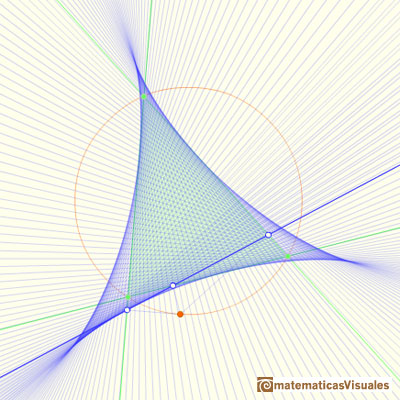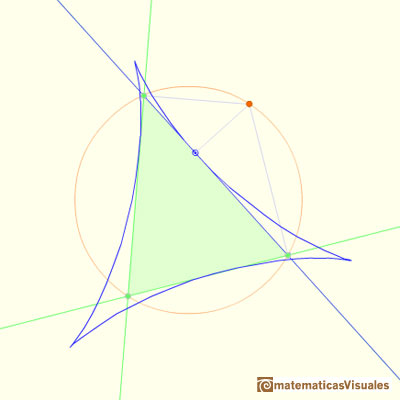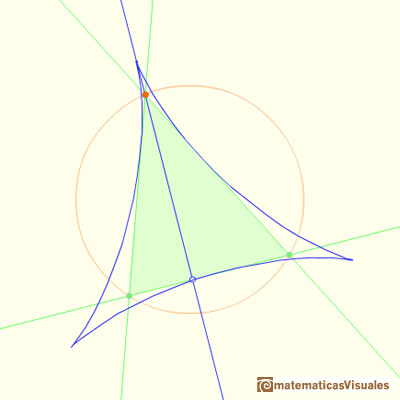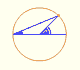Steiner Deltoid and Simson Lines

Steiner proved that the envelope of the Wallace-Simson lines is a curve that has three cuspidal points that is called the Steiner deltoid.The Steiner Deltoid is tangent to the three sides of the triangle.In this case, can you say where is the red point in the circumcircle in relation with some vertex of the triangle?

The Steiner Deltoid is tangent to the three altitudes of the triangle.REFERENCES

Coxeter, H. S. M. and Greitzer, S. L. Geometry Revisited. Washington, DC: Math. Assoc. Amer.
Coxeter, H. S. M. Introduction to Geometry, 2nd ed. New York: John Wiley and sons, 1969.Each point in the circle circunscribed to a triangle give us a line (Wallace-Simson line)Steiner Deltoid and the Morley triangle are related.Central angle in a circle is twice the angle inscribed in the circle.Interactive 'Mostation' of the property of central and inscribed angles in a circle. Case I: When the arc is half a circle the inscribed angle is a right angle.Interactive 'Mostation' of the property of central and inscribed angles in a circle. Case II: When one chord that forms the inscribed angle is a diameter.Interactive 'Mostation' of the property of central and inscribed angles in a circle. The general case is proved.Using a ruler and a compass we can draw fifteen degrees angles. These are basic examples of the central and inscribed in a circle angles property.The three points of intersection of the adjacent trisectors of the angles of any triangle are the vertices of an equilateral triangle (Morley's triangle)Interactive animation about John Conway's beautiful proof of Morley's Theorem